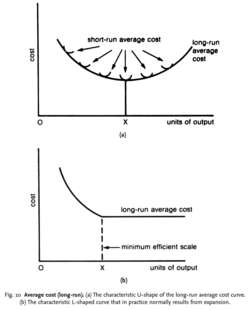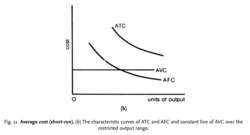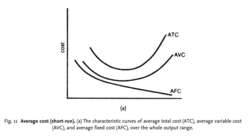# average cost

(redirected from average benefit)
Also found in: Dictionary, Thesaurus, Medical.
Related to average benefit: average cost

## Average cost

In the context of investing, refers to the average cost of shares or stock bought at different prices over time.

## Average Cost

1. A method of determining the value of securities in a tax year. One calculates the average cost by taking the total cost of buying shares in a security and dividing it by the number of shares one owns. The average-cost method is useful especially when the security has fluctuated significantly in price and when the investor has an automatic investment plan.

2. In inventory, a method to determine the value of one unit. It is calculated by dividing the total cost of buying the inventory by the units available for sale. See also: Inventory Valuation.

## average cost

A cost amount calculated by dividing the total cost by the units of production. Thus, if a firm produces 10,000 units of output for a total cost of \$25,000, the average cost of each unit is \$25,000/10,000 units, or \$2.50 per unit. Average cost is made up of costs remaining unchanged throughout a range of output and costs varying directly with output. Firms with the lowest average cost in an industry have a competitive advantage in the event of severe competition and price cutting.

## average cost

the unit cost of a product (total cost divided by number of units produced). Average total cost (ATC) can be split up into average FIXED COST (AFC) and average VARIABLE COST (AVC). AFC declines continuously as output rises, as a given total amount of fixed cost is ‘spread’ over a greater number of units. For example, with fixed costs of £1,000 per year and annual output of 1000 units, fixed costs per unit would be £1, but if annual output rose to 2000 units the fixed cost per unit would fall to 50p. Unit variable cost (AVC) tends to remain constant over the output range.
Collins Dictionary of Business, 3rd ed. © 2002, 2005 C Pass, B Lowes, A Pendleton, L Chadwick, D O’Reilly and M AffersonFig. 10 Average cost (long-run). (a) The characteristic U-shape of the long-run average cost curve.

(b) The characteristic L-shaped curve that in practice normally results from expansion.

## average cost (long-run)

the unit cost (TOTAL COST divided by number of units produced) of producing outputs for plants of different sizes. The position of the SHORT-RUN average total cost (ATC) curve depends on its existing size of plant. In the long run, a firm can alter the size of its plant. Each plant size corresponds to a different U-shaped short-run ATC curve. As the firm expands its scale of operation, it moves from one curve to another. The path along which the firm expands - the LONG-RUN ATC curve - is thus the envelope curve of all the possible short-run ATC curves.

It will be noted that the long-run ATC curve is typically assumed to be a shallow U-shape, with a least-cost point indicated by output level OX. To begin with, average cost falls (reflecting ECONOMIES OF SCALE); eventually, however, the firm may experience DISECONOMIES OF SCALE and average cost begins to rise.

Empirical studies of companies’ long-run average-cost curves, however, suggest that diseconomies of scale are rarely encountered within the typical output ranges over which companies operate, so that most companies’ average cost curves are L-shaped, as in Fig. 10 (b). In cases where diseconomies of scale are encountered, the MINIMUM EFFICIENT SCALE at which a company will operate corresponds to the minimum point of the long-run average cost curve (Fig. 10 (a)). Where diseconomies of scale are not encountered within the typical output range, minimum efficient scale corresponds with the output at which economies of scale are exhausted and constant returns to scale begin (Fig. 10 (b)). Compare AVERAGE COST (SHORT-RUN).Fig. 11 Average cost (short-run). (b) The characteristic curves of ATC and AFC and constant line of AVC over the restricted output range.Fig. 11 Average cost (short-run). (a) The characteristic curves of average total cost (ATC), average variable cost (AVC), and average fixed cost (AFC), over the whole output range.

## average cost (short-run)

the unit cost (TOTAL COST divided by the number of units produced) of producing particular volumes of output in a plant of a given (fixed) size. Average total cost (ATC) can be split up into average FIXED COST (AFC) and average VARIABLE COST (AVC). AFC declines continuously as output rises as a given total amount of fixed cost is ‘spread’ over a greater number of units. For example, with fixed costs of £1,000 per year and annual output of 1,000 units, fixed costs per unit would be £1, but if annual output rose to 2,000 units, the fixed cost per unit would fall to 50 pence - see AFC curve in Fig. 11 (a).

Over the whole potential output range within which a firm can produce, AVC falls at first (reflecting increasing RETURNS TO THE VARIABLE FACTOR INPUT output increases faster than costs), but then rises (reflecting DIMINISHING RETURNS to the variable inputs - costs increase faster than output), as shown by the AVC curve in Fig. 11 (a). Thus the conventional SHORT-RUN ATC curve is U-shaped.

Over the more restricted output range in which firms typically operate, however, constant returns to the variable input are more likely to be experienced, where, as more variable inputs are added to the fixed inputs employed in production, equal increments in output result. In such circumstances, AVC will remain constant over the whole output range, as in Fig. 11 (b), and as a consequence ATC will decline in parallel with AFC. Compare AVERAGE COST (LONG-RUN). See LOSS, LOSS MINIMIZATION.

Collins Dictionary of Economics, 4th ed. © C. Pass, B. Lowes, L. Davies 2005
References in periodicals archive ?
We closely examined how and the extent to which states and their neighbors changed their welfare benefit levels relative to the national average benefit level.
This is projected to lead to a reduction in average benefits equivalent to 5 to 6 percentage points of GDP.
Sixth, the average benefits safe harbor provides a standard that takes into account the relative level of benefits of one line compared to the level of benefits in other lines.
The average benefit percentage means the average of the benefit percentages calculated separately with regard to each employee in the group.
For the transfer to be worthwhile we want the average benefit to exceed the average cost and therefore:
According to the preamble, Congress was concerned about the economic disadvantage that employers could face if the average benefit percentage test were applied on an employer-wide basis in those situations where the level of benefits varied significantly among the employer's separate lines of business for competitive market reasons.
The average benefit in 2016: \$1,350 per month, or just over \$16,000 annually.
Never mind that the amount of the average benefit is about \$270 a week - an amount some lawmakers drop on a single night out on the town.
The gains are tangibly spelt out in the report with the assertion that the average benefit per linked system is [pounds sterling]12,500 for district councils, rising to over [pounds sterling]22,000 for Unitaries and London Boroughs.
An effective way to communicate the value of enrollment meetings is to show statistics about a company's average benefit plan participation rates.
If each is entitled to the average benefit, their total annual Social Security benefit will be \$20,856 (\$12,648 for the husband and \$8,208 for the wife).
For members not covered under Social Security, the average benefit percentage grew from 2.32 percent to 2.35 percent during the two years.

Site: Follow: Share:
Open / Close